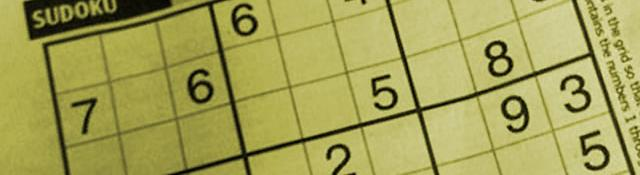## Grid detection

Series: SuDoKu Grabber in OpenCV:

In this post,we 'll look at detecting a SuDoKu puzzle. This include all preprocessing done on the image: filtering the image to ensure we're not affected too much by noise. Also, segmenting the image is dealt with here. I've used a weird segmentation approach, so you might want to have a look at that. By the end of this post, you'll have several possible lines that describe the puzzle grid.

## Getting started

Start by creating a new project in your IDE. If you're not sure how that is done, have a look at the Getting started with OpenCV guide.

Also, I'll use OpenCV's C++ interface. So make sure you have at least OpenCV 2.0 installed on your computer.

Link your project to the OpenCV library files and include the following in your main file:

```#include <cv.h>
#include <highgui.h>

int main()
{
return 0;
}
```

For now, we'll use a static image for detecting a puzzle. So we load an image:

```int main()
{
Mat sudoku = imread("sudoku.jpg", 0);
```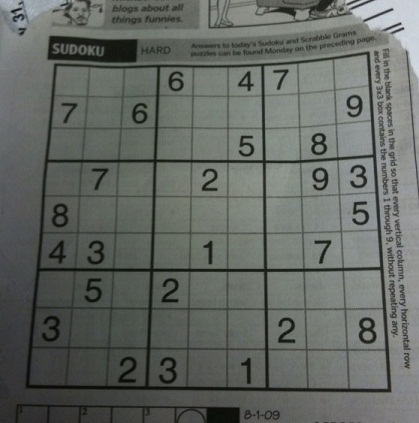An image with a sudoku puzzle

Note that we load the image in grayscale mode. We don't want to bother with the colour information, so just skip it. Next, we create a blank image of the same size. This image will hold the actual outer box of puzzle:

```    Mat outerBox = Mat(sudoku.size(), CV_8UC1);
```

## Preprocessing the image

Blur the image a little. This smooths out the noise a bit and makes extracting the grid lines easier.

```    GaussianBlur(sudoku, sudoku, Size(11,11), 0);
```

With the noise smoothed out, we can now threshold the image. The image can have varying illumination levels, so a good choice for a thresholding algorithm would be an adaptive threshold. It calculates a threshold level several small windows in the image. This threshold level is calculated using the mean level in the window. So it keeps things illumination independent.

```    adaptiveThreshold(sudoku, outerBox, 255, ADAPTIVE_THRESH_MEAN_C, THRESH_BINARY, 5, 2);
```

It calculates a mean over a 5x5 window and subtracts 2 from the mean. This is the threshold level for every pixel.

Since we're interested in the borders, and they are black, we invert the image outerBox. Then, the borders of the puzzles are white (along with other noise).

```    bitwise_not(outerBox, outerBox);
```

This thresholding operation can disconnect certain connected parts (like lines). So dilating the image once will fill up any small "cracks" that might have crept in.

```    Mat kernel = (Mat_<uchar>(3,3) << 0,1,0,1,1,1,0,1,0);
dilate(outerBox, outerBox, kernel);
```

Note that I've used a plus shaped structuring element here (the kernel matrix).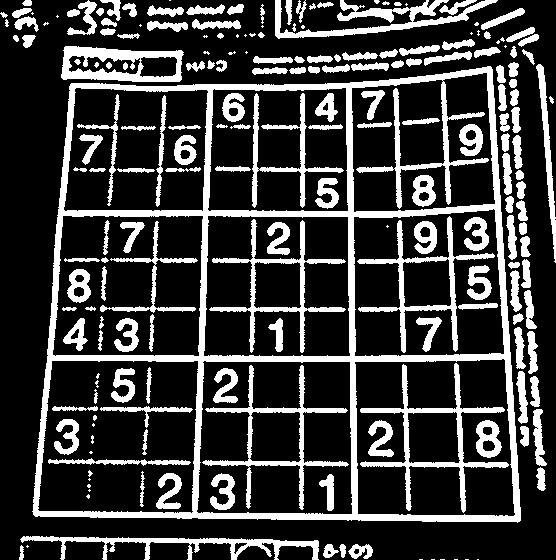After inverting and dilating the puzzle

## Finding the biggest blob

For this project, I didn't want to use any library for blobs. So I made a little hack for detecting blobs. If you want, you can use cvBlobsLib.

Here's the technique I use. First, I use the floodfill command. This command returns a bounding rectangle of the pixels it filled. We've assumed the biggest thing in the picture to be the puzzle. So the biggest blob should have be the puzzle. Since it is the biggest, it will have the biggest bounding box as well. So we find the biggest bounding box, and save the location where we did the flood fill.

```    int count=0;
int max=-1;

Point maxPt;

for(int y=0;y<outerBox.size().height;y++)
{
uchar *row = outerBox.ptr(y);
for(int x=0;x<outerBox.size().width;x++)
{
if(row[x]>=128)
{

int area = floodFill(outerBox, Point(x,y), CV_RGB(0,0,64));

if(area>max)
{
maxPt = Point(x,y);
max = area;
}
}
}

}
```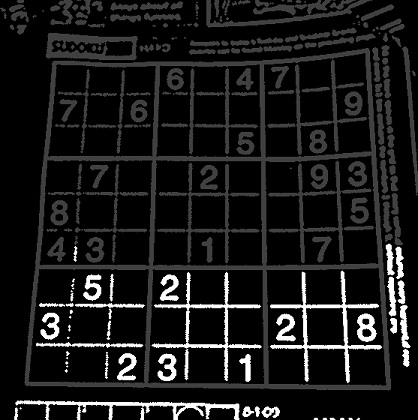Flood filling each blob (in progress)

We iterate through the image. The >=128 condition is to ensure that only the white parts are flooded. Whenever we encounter such a part, we flood it with a dark gray colour (gray level 64). So in the future, we won't be reflooding these blobs. And whenever we encounter a big blob, we note the current point and the area it has.

Now, we have several blobs filled with a dark gray colour (level 64). And we also know the point what produces a blob with maximum area. So we floodfill that point with white:

```    floodFill(outerBox, maxPt, CV_RGB(255,255,255));
```

Now, the biggest blob is white. We need to turn the other blobs black. We do that here:

```    for(int y=0;y<outerBox.size().height;y++)
{
uchar *row = outerBox.ptr(y);
for(int x=0;x<outerBox.size().width;x++)
{
if(row[x]==64 && x!=maxPt.x && y!=maxPt.y)
{
int area = floodFill(outerBox, Point(x,y), CV_RGB(0,0,0));
}
}
}
```

Wherever a dark gray point is enountered, it is flooded with black, effectively "hiding" it.

Because we had dilated the image earlier, we'll "restore" it a bit by eroding it:

```    erode(outerBox, outerBox, kernel);
imshow("thresholded", outerBox);
```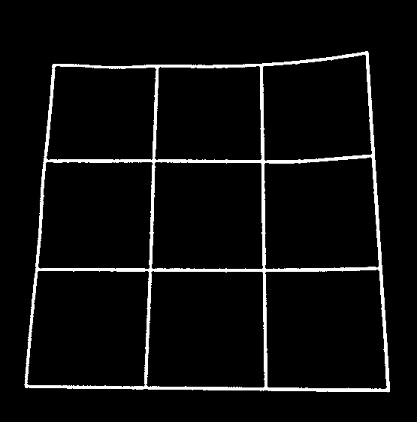The biggest blob, after morphological erosion

## Detecting lines

At this point, we have a single blob. Now its time to find lines. This is done with the Hough transform. OpenCV comes with it. So a line of code is all that's needed:

```    vector<Vec2f> lines;
HoughLines(outerBox, lines, 1, CV_PI/180, 200);
```

For now, we'll draw each line. Just to see if the results too now are good enough or not:

```    for(int i=0;i<lines.size();i++)
{
drawLine(lines[i], outerBox, CV_RGB(0,0,128));
}
```

Where, the drawLine function is:

```void drawLine(Vec2f line, Mat &img, Scalar rgb = CV_RGB(0,0,255))
{
if(line!=0)
{
float m = -1/tan(line);

float c = line/sin(line);

cv::line(img, Point(0, c), Point(img.size().width, m*img.size().width+c), rgb);
}
else
{
cv::line(img, Point(line, 0), Point(line, img.size().height), rgb);
}

}
```

This function takes a line in the normal form (a distance from the original and angle with the x-axis). Then, if the line is vertical (infinite slope), it draws the line appropariately. If not, it finds two points on the line and draws a line accordingly.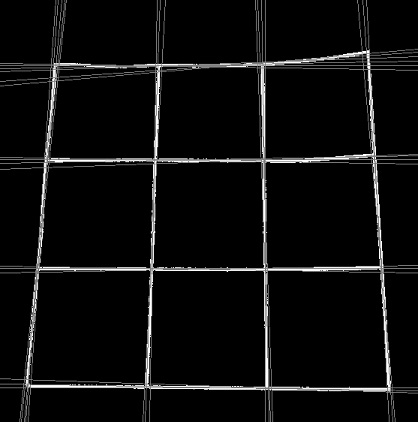Lines detected by the Hough transform

As you can see, each physical line has several possible approximations. This is usually because the physical line is thick. So, just these lines aren't enough for figuring out where the puzzle is located. We'll have to do some math with these lines. But we'll do that in the next post. I think this one has been long enough.

## Summary

Today, we implemented the first half of the SuDoKu grabber. We've been able to detect the physical borders of the puzzle till now, but the results aren't usable directly. We'll do a little math with them and fix that next.

## More in the series

This tutorial is part of a series called SuDoKu Grabber in OpenCV:

Utkarsh Sinha created AI Shack in 2010 and has since been working on computer vision and related fields. He is currently at Microsoft working on computer vision.